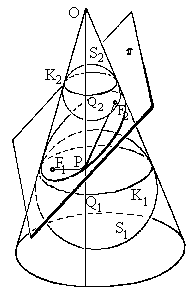## Conic SectionsAs is well known there are three (proper) conic sections: ellipse, parabola and hyperbola. They are so called because one way to obtain them is to cut with a plane through a (circular) cone. Depending on the relative position of the plane and the cone, the intersection will present one of the three conic sections. (But there are also exceptional cases.)Ellipse is known by its focal definition: it's a locus of all points P in the plane the sum of whose distances from two fixed points F1 and F2, the foci, has a constant value. In 1822, the Belgian mathematician G. P. Dandelin gave a beatiful proof of the following result:

When a conic section is a finite shape it's always an ellipse.

That such a simple proof of the result known yet to ancient Greeks was discovered in the 19th century is nothing short of wonderful.

### Proof

Imagine first a huge sphere S1 inside the cone. Let the sphere be so big as to not touch the cutting plane. Assume it touches the cone over the circle K1. Shrink the sphere S1 (suck it into the cone) until it touches the plane at point F1. From the other side, near the vertex O, imagine another sphere S2, now so small as not to touch the plane. Let it touch the cone over a circle K2. Let S2 grow until it finally touches the plane at F2. As you may have guessed, F1 and F2 are the two foci we need to establish that the conic section is indeed an ellipse.

Observe first that the circles K1 and K2 are parallel. Therefore any straight line on the surface of the cone passing through its vertex will cut a segment Q1Q2 of constant length between the two circles.

Next, let P be a point on the conic section. Draw a line OQ1Q2 through P. Since Both PQ1 and PF1 are tangent to S1, we have PQ1 = PF1. Similarly, PQ2 = PF2 because both PQ2 and PF2 are tangent to S2. Therefore, PF1 + PF2 = PQ1 + PQ2. But the latter has just been shown to be constant. Q.E.D.

(The spheres S1 and S2 are now known as Dandelin's spheres. Similar proofs and spheres are constructed for hyperbola and parabola.)

Then there is a way to visualize conic sections. Watch the shadow thrown by a ball when a light source moves around it. The border of the shadow is liable to be one of the three conic sections.### References

1. R.Courant and H.Robbins, What is Mathematics?, Oxford University Press, 1996
2. D.Hilbert and S.Cohn-Vossem, Geometry and Imagination, Chelsea Publishing Co., NY, 1990
3. R.Honsberger, More Mathematical Morsels, MAA, New Math Library, 1991

There is an extensive bibliography of the related materials available online at the exemplary site by Xah Lee.### Remarks on Exceptional Sections

First of all, a cone is characterized by three elements: its vertex, its axis of symmetry and its generating line. When the cutting plane is perpendicular to the axis of symmetry, the elliptic section reduces to its particular case, a circle. A circle is an ellipse, for which two focal points coalesce into one - the center of the circle.

Second of all, three exceptional cases arise when the cutting plane passes through the vertex of the cone. The plane may have no other points in common with the cone, so that the vertex - a single point - should be considered a conic section in its own right. A plane through the vertex that meets a cone in other points may be either tangent to the cone at one of the generating lines or cross the cone at two straight lines that meet at the vertex. Seeing the former case as the limiting configuration of the latter, it's customary to say that in both cases the section consists of two straight lines, that coincide when the plane is tangent to the cone.

The three exceptional cases are known as degenerate conics.

Third of all, the vertex may be moved to infinity. The cone then degenerates into a cylinder, that, besides an ellipse, may have two parallel lines or even an empty set as its sections.70791446